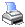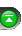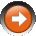﻿ Using [] brackets
 NinjaScript > Educational Resources > Tips > Using [] bracketsIn C#, square brackets represent a way to access values stored within an array. NinjaScript comes with quite a few arrays that we call DataSeries objects which could be accessed with square brackets. DataSeries objects are linked to the underlying bars series in that they hold the same number of values as the number of bars on a chart. For example, to get the close price one bar ago, you would use Close since the value of 1 within the square brackets represents the number of bars ago whose value you wish to reference. As another example, to get the high three bars ago, you would use High.

 double close1 = Close; // gets the close price one bar ago double high3 = High; // gets the high of three bars ago double low = Low; // results in compile error. Low is an array, and can't be accessed directly. It should be Low[n Bars ago].

Many of NinjaTrader's indicators store their values in plots. Plots are essentially DataSeries objects and to retrieve values from them you need to specify which value you want to access. In most cases, you'd like the current value, so you could use SMA(14), not just SMA(14). SMA(14) is the DataSeries, and you can't access it directly. Using SMA(14) retrieves the part of the DataSeries you're interested in--the most current value.

 double SMA_current = SMA(14); // gets the current value of the SMA double SMA_1 = SMA(14); // gets the SMA value one bar ago double SMA_value = SMA(14); // results in compile error. SMA(14) is a DataSeries and the variable SMA_value of type double can't hold a DataSeries.

Most of the time, you need an index value (number in the square brackets), but there are also cases when you need to use the DataSeries instead. CrossAbove() and CrossBelow() are two key examples. If you look at the reference page for CrossAbove(), the two method signatures (overloads) look like this:

 CrossAbove(IDataSeries series1, double value, int lookBackPeriod) CrossAbove(IDataSeries series1, IDataSeries series2, int lookBackPeriod)

This means the first variable must always be a DataSeries object, and the second variable can be either another DataSeries or a double value (100, 70.25, etc). To specify a DataSeries object, you can just leave off the square brackets. For example if(CrossAbove(SMA(14), SMA(28), 1)) checks if the 14 period SMA has crossed above the 28 period SMA within the last bar. if(CrossAbove(SMA(14), SMA(28), 1)) would give you a compile error because it expects a DataSeries as input,not a double value (which is returned when an index is present).

 if (CrossAbove(SMA(14), SMA(28), 1)) // works fine if (CrossAbove(SMA(14), 1000, 1)) // works fine, this uses a double for the second argument. See the above overload. if (CrossAbove(SMA(14), SMA(28), 1)) // compile error: SMA(14) is a double, not a DataSeries if (CrossAbove(SMA(14), SMA(28), 1)) // would work fine with a DataSeries as first argument and a double as the second argument